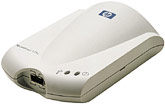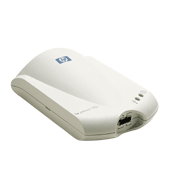criggie.org.nz

 News Images MP3 Humour Email Us Webmail Links Stuff from: Debbie Criggie Daniel Luke Katherine Gladys

# HP Jetdirect 175x USB/EthernetThese things are absolutely brilliant. Any ethernet network with more than a totally minor printing requirement should look at a shared printer with network capabilities. This item permits many kinds of USB printer to listen to the network for jobs, rather than depending on a workstation being up.

## nmap -O usblaser

```socks:/tmp# nmap -O usblaser

Starting nmap 3.27 ( www.insecure.org/nmap/ ) at 2003-09-22 15:09 NZST
Interesting ports on usblaser.avonside.school.nz (192.168.1.44):
(The 1618 ports scanned but not shown below are in state: closed)
Port       State       Service
23/tcp     open        telnet
80/tcp     open        http
280/tcp    open        http-mgmt
515/tcp    open        printer
9100/tcp   open        jetdirect
No exact OS matches for host (If you know what OS is running on it, see http://w
ww.insecure.org/cgi-bin/nmap-submit.cgi).
TCP/IP fingerprint:
SInfo(V=3.27%P=i686-pc-linux-gnu%D=9/22%Time=3F6E680A%O=23%C=1)
TSeq(Class=RI%gcd=1%SI=C3D3%IPID=I%TS=2HZ)
TSeq(Class=RI%gcd=1%SI=D402%IPID=I%TS=2HZ)
TSeq(Class=RI%gcd=1%SI=D024%IPID=I%TS=2HZ)
T1(Resp=Y%DF=N%W=2000%ACK=S++%Flags=AS%Ops=MNWNNT)
T2(Resp=N)
T3(Resp=Y%DF=N%W=2000%ACK=O%Flags=A%Ops=NNT)
T4(Resp=Y%DF=N%W=2000%ACK=O%Flags=R%Ops=)
T5(Resp=Y%DF=N%W=0%ACK=S++%Flags=AR%Ops=)
T6(Resp=Y%DF=N%W=0%ACK=O%Flags=R%Ops=)
T7(Resp=Y%DF=N%W=0%ACK=S%Flags=AR%Ops=)
PU(Resp=Y%DF=N%TOS=0%IPLEN=38%RIPTL=148%RID=F%RIPCK=0%UCK=0%ULEN=134%DAT=E)

Uptime 0.003 days (since Mon Sep 22 15:05:06 2003)

Nmap run completed -- 1 IP address (1 host up) scanned in 36.634 seconds
```
• 10/100 ethernet
• USB 1.1 Port
• Power is 13 Volts DC, 300 milliamp, with TIP as POSITIVE
• and one TEST button

## Documentation